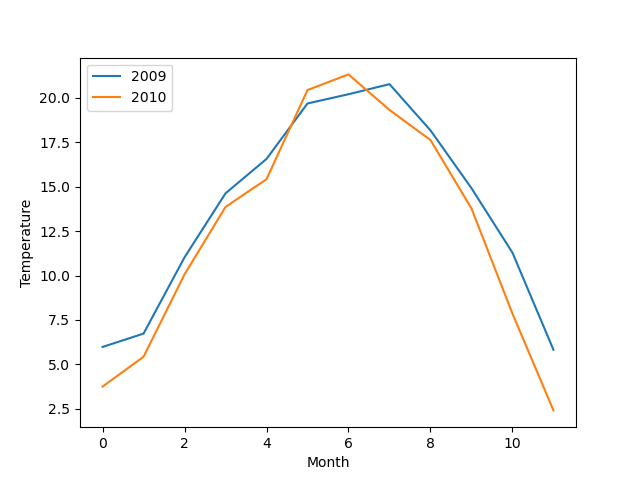# Multiple line plots in Matplotlib

By Martin McBride, 2022-06-18
Tags: numeric python multiple plots
Categories: matplotlib numpyIn this section, we will learn how to show more than one data set on the same line plot.

We will use the monthly temperature data for both 2009 and 2010 to create line plot with both data sets.

## Line plot with two data sets

Here is a line plot showing the temperatures for 2009 and 2010:And here is the code to create it:

import matplotlib.pyplot as plt
import csv

with open("2009-temp-monthly.csv") as csv_file:
temperature_2009 = [x for x in csv_reader]

with open("2010-temp-monthly.csv") as csv_file:
temperature_2010 = [x for x in csv_reader]

months = range(12)

plt.xlabel("Month")
plt.ylabel("Temperature")

plt.plot(months, temperature_2009, label="2009")
plt.plot(months, temperature_2010, label="2010")
plt.legend(loc="upper left")
plt.show()


The code is here on github, in the file 2year-lineplot-monthly-temperatures.py.

This is similar to the line plots we have seen before, with a couple of differences.

Firstly, we read two sets of data:

• 2009-temp-monthly.csv is read into the list temperature_2009.
• 2010-temp-monthly.csv is read into the list temperature_2010.

Secondly, we call plt.plot twice, to create the two line plots:

plt.plot(months, temperature_2009, label="2009")
plt.plot(months, temperature_2010, label="2009")


Matplotlib automatically gives the second plot a different colour.

Each plot is given a label. This is used to display the legend in the top left corner of the plot, indicating that the blue curve represents 2009, and the orange curve represents 2010.

The other difference is that we are dealing with monthly data, rather than daily data, so there are only 12 data values in each series. This means that we only need 12 x-values, one for each moth, obtained using:

months = range(12)